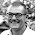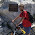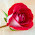Support us .Net Basics C# SQL ASP.NET ADO.NET MVC Slides C# Programs Subscribe Buy DVD

### Part 7 - C# Tutorial - Datatype conversions

Suggested Videos
Part 4 - String type
Part 5 - Operators
Part 6 - Nullable Types

In this video, we will discuss
1. Implicit conversions
2. Explicit Conversions
3. Difference between Parse() and TryParse()

Implicit conversion is done by the compiler:
1. When there is no loss of information if the conversion is done
2. If there is no possibility of throwing exceptions during the conversion

Example: Converting an int to a float will not loose any data and no exception will be thrown, hence an implicit conversion can be done.

Where as when converting a float to an int, we loose the fractional part and also a possibility of overflow exception. Hence, in this case an explicit conversion is required. For explicit conversion we can use cast operator or the convert class in c#.

Implicit Conversion Example
using System;
class Program
{
public static void Main()
{
int i = 100;

// float is bigger datatype than int. So, no loss of
// data and exceptions. Hence implicit conversion
float f = i;

Console.WriteLine(f);
}
}

Explicit Conversion Example
using System;
class Program
{
public static void Main()
{
float f = 100.25F;

// Cannot implicitly convert float to int.
// Fractional part will be lost. Float is a
// bigger datatype than int, so there is
// also a possiblity of overflow exception
// int i = f;

// Use explicit conversion using cast () operator
int i = (int)f;

// OR use Convert class
// int i = Convert.ToInt32(f);

Console.WriteLine(i);
}
}

Difference between Parse and TryParse
1. If the number is in a string format you have 2 options - Parse() and TryParse()
2. Parse() method throws an exception if it cannot parse the value, whereas TryParse() returns a bool indicating whether it succeeded or failed.
3. Use Parse() if you are sure the value will be valid, otherwise use TryParse()

1.namespace tryparse
{
class Program
{
static void Main(string[] args)
{
string value = "200t";
int r=0;
bool enternumber = int.TryParse(value, out r);

if (enternumber)
{

Console.WriteLine( r);
}
else
{
Console.WriteLine("please enter the correct number " + r);
}
}

}
}

2.It has been a long time since I have done any programming. These tutorials are nice to have to get me started again. Thank You.

When I was messing with the explicit conversion using your examples one thing interesting I noticed, when converting from float to integer ( int i = Convert.ToInt16(f); ) was that the integer was rounded UP if the fraction was larger than 0.5.

When using ( int i = (int)f: ) the fraction was just lost.

I am using MS Visual studio 2012 and don't have anything else to compare to. Just figured this was something worth mentioning.

Again thanks for the tutorials.

Rick

3.These are the excellent videos and any one can understand!!! Thanks Venkat.
Sajjan

4.Hi Venkat thanks a lot... From Barcelona... Muchas gracias

5.thanks venkat sir ,all videos are very easy to understand

6.Excellent blog as learning platform!

7.sir please solve this error
using System;
//using System.Collections.Generic;
//using System.Linq;
//using System.Text;

namespace operatorsproject3
{
class Program
{
static void Main(string[] args)
{
int num1;
int num2;
string operand;

Console.WriteLine("enter the first number ");

Console.WriteLine("enter the operand: +, -, *, / ");

Console.WriteLine("please enter the second number");

switch (operand)
{
case"+":
answer = num1 + num2;
break;

case"-":
answer = num1 - num2;
break;

case "*":
answer = num1 * num2;
break;

case "/":
answer = num1 / num2;
break;

default:
answer = Convert.ToString(Console.WriteLine("enter wrong value"));
break;

}

Console.WriteLine("Answer {0} {1} {2} {3} {4}", num1, operand, num2 ,"=", answer);

}
}
}

1.using System;
namespace TryParse
{
class parseProgram
{
static void Main()
{
int num1;
int num2;
string operand;
Console.WriteLine("Enter First Number");
Console.WriteLine("Enter Second Number");

Console.WriteLine("Enter Operand");

switch (operand)
{
case"+": answer = num1 + num2;
break;
case "-": answer = num1 - num2;
break;
case "*":
answer = num1 * num2;
break;
case "/":
answer = num1 / num2;